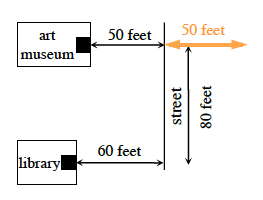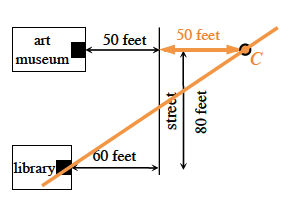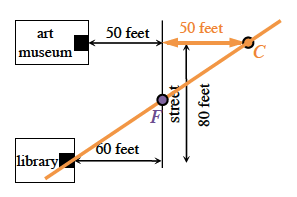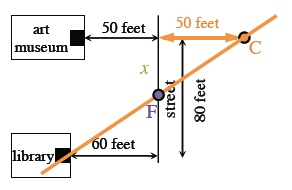### Home > CCG > Chapter 7 > Lesson 7.2.1 > Problem7-56

7-56.

Sandra wants to park her car so that she optimizes the distance she has to walk to the art museum and the library. That is, she wants to park so that her total distance directly to each building is the shortest. Find where she should park.Reflect the $50$ feet line across the street. Added to diagram, orange horizontal line segment, right of the vertical street line segment, at the same height as the art museum.

Draw a straight line from the library to point $C$Added to diagram, point c, at end of horizontal segment, & diagonal line segment connecting point, c, to library.

Point $F$ is where Sandra should park her car. Added to diagram, point labeled, f, where the diagonal segment, crosses the vertical street line segment.

Write an equation based on similar triangles to calculate the length $x$. If the length of the entire segment is $80$ and one piece is $x$, how can you label the other piece?  Added to diagram, the label, x, along the vertical street segment, between point, f, & the horizontal segment from the art museum.